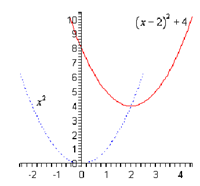## Using transformation to sketch the graph, Algebra

Assignment Help:

Using transformation to sketch the graph

f ( x ) = (x - 2)2  + 4

Solution

In this part it looks as the base function is x2 and it looks as will be shift it to the right by 2  (as c = -2 ) and up by 4 (since k = 4 ).  Following is the sketch of this function.3x-2(4x+9)=-58

#### Cyckic group, which of the following are cyclic group G1= G2= G3= G4= G...

which of the following are cyclic group G1= G2= G3= G4= G5={6n/n belong to z}

y2/3(y4/3\y1/3

#### Horizontal shifts, Horizontal Shifts These are quite simple as well tho...

Horizontal Shifts These are quite simple as well though there is one bit where we have to be careful. Given the graph of f ( x ) the graph of g ( x ) = f ( x + c ) will be t

#### Determine the two zeroes - factor theorem, Given that x=2 is a zero of P ( ...

Given that x=2 is a zero of P ( x ) = x 3 + 2x 2 - 5x - 6 determine the other two zeroes. Solution Firstly, notice that we actually can say the other two since we know th

#### Polynomials and factoring, how can you model the addition of polynomials wi...

how can you model the addition of polynomials with algebra tiles

#### , find the inverse function f(x)=log12(x

find the inverse function f(x)=log12(x)

#### Solve the equation, 3^x + 3^(x^1/2) - 90 =0

3^x + 3^(x^1/2) - 90 =0

#### D=rt word problem solver!, Carl and his dad I stonecarvers. Carl can cut th...

Carl and his dad I stonecarvers. Carl can cut the stone in five days. With his father''s help he can carve it in two days. How long would take his father to carve it alone. Bob c

#### Solve each equation, 5(4a+1)+6=-(-11a+1)+6a

5(4a+1)+6=-(-11a+1)+6a